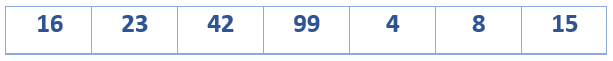Home Arrays Write a program to rotate an array.

# Write a program to rotate an array.

0 comment

Question: Write a program to rotate an array arr[], having a size ‘n’ by ‘d’ elements?

Input: arr[] = { 4, 8, 15, 16, 23, 42, 99 }, d = 3
Output: 16, 23, 42, 99, 4, 8, 15

Let us suppose our array is in this way:On rotating the array by 3 elements, it should look like:To solve the above problem, we can adopt this following technique-

• Create a function that rotates the entire array by one element at a time.
• To rotate by one, store arr in a temporary variable temp, move arr to arr, arr to arr …and finally temp to arr[n-1]
• Create a function rotate(arr[], d, n) that calls the above function ‘d’ number of times.

Here is an implementation of the above algorithm:-

#include<stdio.h>

//function to rotate the array by one element
void leftRotatebyOne(int arr[], int n)
{
int i, temp;
temp = arr;

//swap all the elements
for (i = 0; i < n-1; i++)
arr[i] = arr[i+1];

arr[i] = temp;
}

/*Function to left rotate arr[] of size n by d*/
void leftRotate(int arr[], int d, int n)
{
int i;

//call the function to rotate by 1, d number of times
for (i = 0; i < d; i++)
leftRotatebyOne(arr, n);
}

//utility function to print an array
void printArray(int arr[], int size)
{
int i;
for(i = 0; i < size; i++)
printf("%d ", arr[i]);
}

//Driver program to test above functions
int main(void)
{
int arr[] = {4, 8, 15, 16, 23, 42, 99};

leftRotate(arr, 3, 7);
printArray(arr, 7);

return 0;
}


Time Complexity: O(n * d)
Space Complexity: O(1)